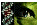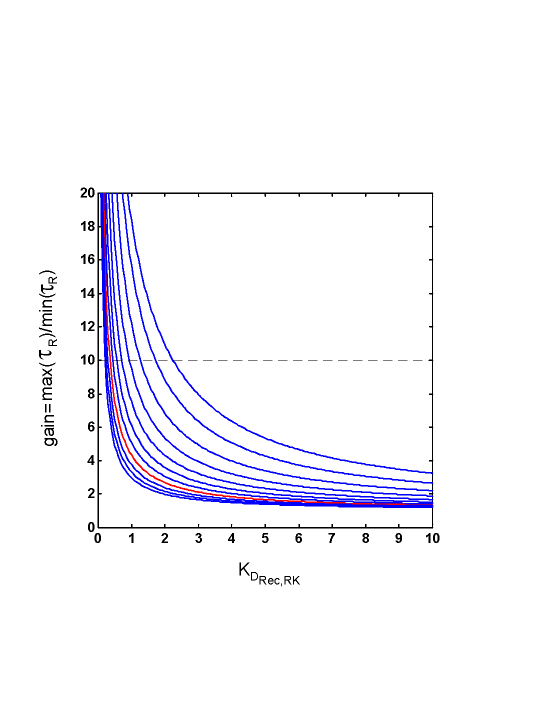Figure 8 of Hamer, Mol Vis 2000; 6:265-286.

Figure 8. The need for a large front-end Ca++-mediated gain severely constrains RecRK parameters

The relationship between R* lifetime gain change and the dissociation constant for the interaction between Ca++-Rec complex and RK (i.e., KD = q4/q3 in Eq. 8, A10). The gain change (ordinate) is defined as the ratio of τRdark (theoretical value of τR when Ca++ = cdark = 0.3 μM) to τRminR when Ca++ = cmin = 0.02 μM). The curves shown correspond to a family of KRec,Ca values ranging from 0.2 to 1.2 μM (from top to bottom, in 0.1 μM increments). The red curve is for KRec,Ca = 0.9 μM, a value obtained by Klenchin et al. . The horizontal dashed line marks a gain of 10. See text for details.

Note that KD must be very small if other RecRK- and Ca++ parameters are set to values estimated in the literature. In this example, Rectot = 34 μM  and the Hill coefficient, w = 2 . For KRec,Ca = ~0.9 μM (red curve) , KD must be less than ~0.25 μM in order to get a gain change of 10x. This value for KD is ~14 times less than the value estimated by Klenchin et al. (~3.4 μM; ). Analyses show that KD will be even more severely constrained (to even smaller values) if it is assumed that the minimum physiological value for Ca++ is greater than 0.02 μM, as estimated in some studies [33,63-65].

Not only is KD restricted to small values, but, due to the steepness of the functions in the region of high gain values (10x or more), the overall range of permissible values is highly restricted. Hence, for a given KRec,Ca value, only a very restricted range of (small) values for KD are permissible in order to achieve the empirically observed gain changes.Hamer, Mol Vis 2000; 6:265-286 <http://www.molvis.org/molvis/v6/a36/>
©2000 Molecular Vision <http://www.molvis.org/molvis/>
ISSN 1090-0535